# Physics - Classical Mechanics - Forced Oscillation and Resonance

in STEMGeeks2 years ago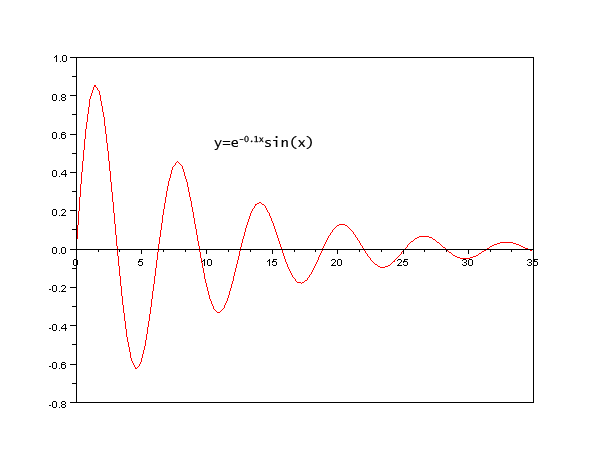[Image1]

## Introduction

Hey it's a me again @drifter1!

Today we continue with Physics, and more specifically the branch of "Classical Mechanics" in order to get into Forced Oscillation and Resonance.

So, without further ado, let's get straight into it!

## Forced Oscillation

Continuing on from the damped oscillator discussed last time, it's possible to add a so called harmonic driving force, that causes the system to oscillate "by force". Such an oscillation is known as forced oscillation.

### Differential Equation

The differential equation that describes the motion of a forced oscillator, is shown below.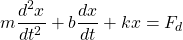where the driving (external) force Fd is of the form: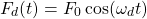The ωd in this equation is called the driving frequency (or frequency of the external force), and isn't necessarily that same as the natural frequency of oscillation (ω0) nor the oscillation (ω) that the damping oscillator has without any external force applied (free system oscillation frequency).

### Amplitude

At first, when the damped oscillator is forced through an external driving force, the motion is chaotic (transient state). After some time has passed it reaches a steady state, where the motion is then periodic: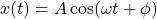Let's skip the proof, and directly state that the amplitude is then given by: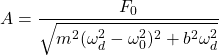Thus, as the frequency of the driving force (ωd) approaches the natural frequency of the system (ω0), the amplitude of the oscillations increases. The amplitude is maximum when ωd0.

### Resonance

Continuing on from that last statement, when ωd0, we say that the system is resonating. Resonance is an interesting phenomenon, and even though it's simple, it can still be critical in lots of situations.

Near resonance, the damping should not be ignored, because as the amplitude reaches infinity the damping constant b reaches zero, which is not physical. Of course, infinite amplitude never occurs in practice, but as the amplitude reaches it's peak there may occur non-linearities in the system (large oscillations) that can be quite catastrophic.

## Final words | Next up

And this is actually it for today's post!

Next time we will get into Exercises on the topics of Damped and Forced Oscillation...

See ya!Keep on drifting!

Posted with STEMGeeks

Sort:
2 years ago Reveal Comment